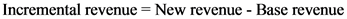# When evaluating a special order. management should:

When evaluating a special order, management should: Only accept the order if the incremental revenue exceeds all
product costs. Only accept the order if the incremental revenue exceeds fixed
product costs. Only accept the order if the incremental revenue exceeds total
variable product costs. Only accept the order if the incremental revenue exceeds full
absorption product costs. Only accept the order if the incremental revenue exceeds regular
sales revenue.

General guidance

Concepts and reason
Cost: Cost is any value spent to produce a product or to render any service. The types of costs are fixed cost and variable cost.
Fixed Cost: Fixed cost is a cost that remains same irrespective of the increase or decrease in the value of goods or any services rendered. It is the cost paid by the company that does not depend on the activities concerned with the business.
Variable Cost: Variable cost is a cost that varies according to the output produced or any service rendered. It is the cost paid by the company that depends on the activities concerned with the business.
Sales revenue: The amount earned by selling goods or services is known as sales revenue. This is the main source of revenue for a company. This is usually referred to as gross sales revenue. Gross sales revenue less returns, allowances, and discounts are known as net sales.

Fundamentals

CVP Analysis: Cost Volume Profit analysis is generally termed as CVP analysis. It determines the effect of operating income and net income due to change in the cost and output. In CVP variable cost per unit is constant and total fixed cost is constant.
Incremental revenue: Incremental revenue is the revenue earned by sale of additional quantity. The formula for calculating incremental revenue is

Product costs: Product costs are the costs incurred to manufacture a product. It includes prime costs and factory overheads.
Fixed product cost: Fixed product cost consists of all the fixed cost incurred up to production
Total variable product cost: Total variable product cost is the cost associated with the product consisting of all variable cost till the manufacture of product.

Step-by-step

Step 1 of 2

The product costs are divided into two different costs namely, fixed cost and variable cost. As per cost-volume-profit analysis, total fixed cost remains constant even if the volume of production changes and fixed cost per unit changes. But, the variable cost per unit remains constant even if the volume of production changes and the total variable cost changes.

The cost and its effect should be analysed for every process. Here, the cost denotes both fixed and variable cost and the effect denotes the change in the costs. Only then, an order can be accepted.

The following statement is not correct:
Variable cost per unit changes and fixed cost per unit remains same with any production level.
The correct statement is variable cost per unit remains constant and fixed cost per unit changes with the changes in the level of production.

Evaluate the special order and the resulting change when the order is accepted.

Step 2 of 2

When evaluating a special order, management should accept when it is profitable and earns revenue than the revenue of the current situation.
Variable cost changes in case of increase in production. Thus, when evaluating a special order, management should only accept the order if the incremental revenue exceeds total variable product costs.

The management should only accept the order if the incremental revenue exceeds total variable product costs.

When evaluating a special order, management should also evaluate the effects of such order. When an order is accepted, it increases the existing production level and thus involves the change in the cost. As stated, fixed cost remains same even if production changes. But variable cost changes with the change in production. Therefore, the management should only accept the order if the incremental revenue exceeds total variable product costs.

Absorption cost includes both fixed and variable cost. So it is wrong if absorption cost is considered.
Regular sales revenue should also eliminated for considering the revenue as the variable cost per unit may change in the upcoming order.